Example Questions

1 2 4 Next →

Example Question #25 : How To Find X Or Y Intercept

Where does the line given by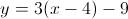intercept the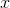-axis?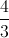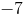Explanation:

First, put in slope-intercept form.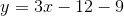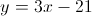To find the-intercept, set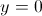and solve for.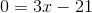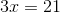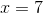Example Question #221 : Coordinate Geometry

Where does the graph of 2x + 3y = 15 cross the x-axis?

(-7.5, 0)

(0, 0)

(7.5, 0)

(0, -5)

(0, 5)